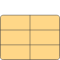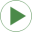# SynchronousMachineData

Computes machine parameter from usual datasheet# Information

This information is part of the Modelica Standard Library maintained by the Modelica Association.

The parameters of the synchronous machine model with electrical excitation (and damper) are calculated from parameters normally given in a technical description, according to the standard EN 60034-4:2008 Appendix C.

# Parameters (47)

SNominal Value: Type: ApparentPower (V·A) Description: Nominal apparent power Value: Type: Voltage (V) Description: Nominal stator voltage per phase Value: SNominal / (3 * VsNominal) Type: Current (A) Description: Nominal stator current per phase Value: VsNominal / IsNominal Type: Impedance (Ω) Description: Reference impedance Value: Type: Frequency (Hz) Description: Nominal stator frequency Value: 2 * pi * fsNominal Type: AngularVelocity (rad/s) Description: Nominal angular frequency Value: Type: Current (A) Description: Open circuit excitation current @ nominal voltage and frequency Value: 1 Type: Real Description: Effective number of stator turns Value: sqrt(2) * VsNominal / (omega * Lmd * IeOpenCircuit) Type: Real Description: Stator current / excitation current Value: Type: Real Description: Stator stray inductance per phase (approximately zero impedance) [pu] Value: Type: Real Description: Synchronous reactance per phase, d-axis [pu] Value: Type: Real Description: Synchronous reactance per phase, q-axis [pu] Value: Type: Real Description: Transient reactance per phase, d-axis [pu] Value: Type: Real Description: Subtransient reactance per phase, d-axis [pu] Value: Type: Real Description: Subtransient reactance per phase, q-axis [pu] Value: Type: Time (s) Description: Armature time constant Value: Type: Time (s) Description: Open circuit field time constant Td0' Value: Type: Time (s) Description: Open circuit subtransient time constant Td0'', d-axis Value: Type: Time (s) Description: Open circuit subtransient time constant Tq0'', q-axis Value: Type: Temperature (K) Description: Specification temperature of stator resistance Value: Type: Temperature (K) Description: Reference temperature of stator resistance Value: Type: LinearTemperatureCoefficient20 (¹/K) Description: Temperature coefficient of stator resistance at 20 degC Value: Type: Temperature (K) Description: Specification temperature of (optional) damper cage Value: Type: Temperature (K) Description: Reference temperature of damper resistances in d- and q-axis Value: Type: LinearTemperatureCoefficient20 (¹/K) Description: Temperature coefficient of damper resistances in d- and q-axis Value: Type: Temperature (K) Description: Specification excitation temperature Value: Type: Temperature (K) Description: Reference temperature of excitation resistance Value: Type: LinearTemperatureCoefficient20 (¹/K) Description: Temperature coefficient of excitation resistance Value: xd - x0 Type: Real Description: Main field reactance per phase, d-axis [pu] Value: xq - x0 Type: Real Description: Main field reactance per phase, q-axis [pu] Value: xmd ^ 2 / (xd - xdTransient) Type: Real Description: Excitation reactance [pu] Value: xmd ^ 2 / (xdTransient - xdSubtransient) * (1 - xmd / xe) ^ 2 + xmd ^ 2 / xe Type: Real Description: Damper reactance per phase, d-axis [pu] Value: xmq ^ 2 / (xq - xqSubtransient) Type: Real Description: Damper reactance per phase, d-axis [pu] Value: 2 / (1 / xdSubtransient + 1 / xqSubtransient) / (omega * Ta) Type: Real Description: Stator resistance per phase at specification temperature [pu] Value: (xrd - xmd ^ 2 / xe) / (omega * Td0Subtransient) Type: Real Description: Damper resistance per phase at specification temperature, d-axis [pu] Value: xrq / (omega * Tq0Subtransient) Type: Real Description: Damper resistance per phase at specification temperature, q-axis [pu] Value: xe / (omega * Td0Transient) Type: Real Description: Excitation resistance per phase at specification temperature [pu] Value: Machines.Thermal.convertResistance(rs * ZReference, TsSpecification, alpha20s, TsRef) Type: Resistance (Ω) Description: Stator resistance per phase at TRef Value: x0 * ZReference / omega Type: Inductance (H) Description: Stator stray inductance per phase Value: xmd * ZReference / omega Type: Inductance (H) Description: Main field inductance per phase in d-axis Value: xmq * ZReference / omega Type: Inductance (H) Description: Main field inductance per phase in q-axis Value: (xrd - xmd) * ZReference / omega Type: Inductance (H) Description: Damper stray inductance in d-axis Value: (xrq - xmq) * ZReference / omega Type: Inductance (H) Description: Damper stray inductance in q-axis Value: Machines.Thermal.convertResistance(rrd * ZReference, TrSpecification, alpha20r, TrRef) Type: Resistance (Ω) Description: Damper resistance in d-axis at TRef Value: Machines.Thermal.convertResistance(rrq * ZReference, TrSpecification, alpha20r, TrRef) Type: Resistance (Ω) Description: Damper resistance in q-axis at TRef Value: 3 / 2 * turnsRatio ^ 2 * Machines.Thermal.convertResistance(re * ZReference, TeSpecification, alpha20e, TeRef) Type: Resistance (Ω) Description: Excitation resistance at TRef Value: 1 - xmd / xe Type: Real Description: Stray fraction of total excitation inductance

# Used in Examples (10)SMEE_DOL Modelica.Electrical.Machines.Examples.SynchronousInductionMachines Test example: ElectricalExcitedSynchronousInductionMachine starting direct on lineSMEE_Generator Modelica.Electrical.Machines.Examples.SynchronousInductionMachines Test example: ElectricalExcitedSynchronousInductionMachine as GeneratorSMEE_LoadDump Modelica.Electrical.Machines.Examples.SynchronousInductionMachines Test example: ElectricalExcitedSynchronousInductionMachine with voltage controllerSMEE_Rectifier Modelica.Electrical.Machines.Examples.SynchronousInductionMachines Test example: ElectricalExcitedSynchronousInductionMachine with rectifierSMEE_DOL Modelica.Magnetic.FundamentalWave.Examples.BasicMachines ElectricalExcitedSynchronousInductionMachine starting direct on lineSMEE_Generator_MultiPhase Modelica.Magnetic.FundamentalWave.Examples.BasicMachines Electrical excited multi phase synchronous machine operating as generatorSMEE_Generator Modelica.Magnetic.FundamentalWave.Examples.BasicMachines Electrical excited synchronous machine operating as generatorSMEE_LoadDump Modelica.Magnetic.FundamentalWave.Examples.BasicMachines Test example: ElectricalExcitedSynchronousInductionMachine with voltage controllerSMEE_Rectifier Modelica.Magnetic.FundamentalWave.Examples.BasicMachines Test example: ElectricalExcitedSynchronousInductionMachine with rectifierSMEE_Generator Modelica.Magnetic.QuasiStatic.FundamentalWave.Examples.BasicMachines.SynchronousMachines Electrical excited synchronous machine operating as generator